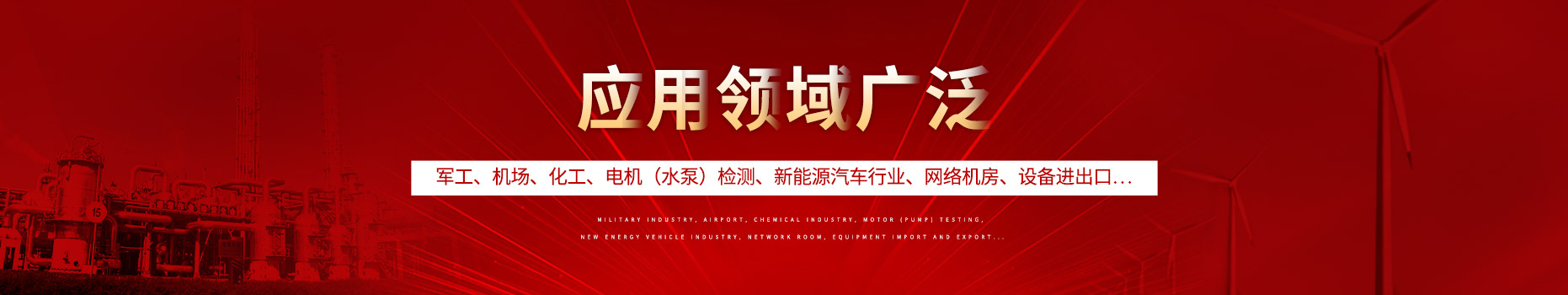400-088-6921155-8888-6921#### 产品分类# 电源电压抑制比

在电子设备中，电源抑制比(power supply rejection ratio,PSRR)是一个经常在电子放大器（特别是运算放大器）或稳压器的规格书出现的参数，用来描述某一设备对电源噪声(来自电源的噪声)的抑制能力。

电源抑制比的定义是电源电压变化做成设备输出的电压相应变化的比例。[因为此比值一般较大，为方便使用，常以分贝（dB）作为单位，理想的设备其PSRR是无限大。PSRR会随频率而改变，规格书一般会以图表展示不同频率下的PSRR，较简单的方式会只标示某特定频率下的PSRR作参考用。

{\displaystyle\mathrm{PSRR}[\mathrm{dB}]=20\log _{10}\left({\Delta V_{\mathrm{supply}}\over{\Delta V_{\mathrm{out}}}}\right){\mbox{dB}}}{\displaystyle\mathrm{PSRR}[\mathrm{dB}]=20\log _{10}\left({\Delta V_{\mathrm{supply}}\over{\Delta V_{\mathrm{out}}}}\right){\mbox{dB}}}

在放大器的情况下，由于整个线路有闭环增益（Av），电源的噪声（△Vsupply）会被放大而得出（△Vout），放大器本身的PSRR会是：

{\displaystyle\mathrm{PSRR}[\mathrm{dB}]=20\log _{10}\left({\Delta V_{\mathrm{supply}}\over{\Delta V_{\mathrm{out}}}}\cdot A_{v}\right){\mbox{dB}}}{\displaystyle\mathrm{PSRR}[\mathrm{dB}]=20\log _{10}\left({\Delta V_{\mathrm{supply}}\over{\Delta V_{\mathrm{out}}}}\cdot A_{v}\right){\mbox{dB}}}

例如一个放大器PSRR=100dB，应用在一个闭环增益=40dB的线路时，整体线路的PSRR就是：

{\displaystyle 100\\mathrm{dB}-40\\mathrm{dB}=60\\mathrm{dB}}{\displaystyle 100\\mathrm{dB}-40\\mathrm{dB}=60\\mathrm{dB}}.

如此，即当电源输出电压为1V时，其输出的噪声就是：

{\displaystyle 1\\mathrm{V}\cdot 10^{\frac{-60}{20}}=.001\\mathrm{V}=1\\mathrm{mV}}{\displaystyle 1\\mathrm{V}\cdot 10^{\frac{-60}{20}}=.001\\mathrm{V}=1\\mathrm{mV}}

### 相关新闻

##### 联系方式

15588886921

400热线

400-0886921

2466458158@qq.com

##### 二维码